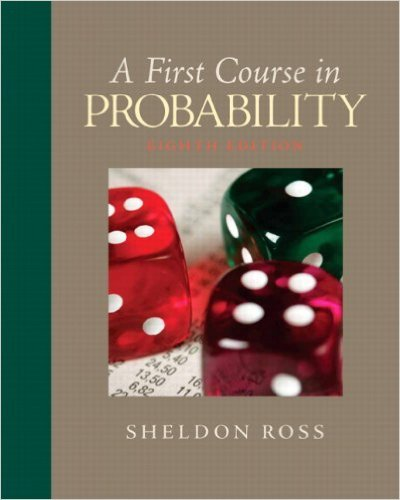×
Get Full Access to First Course In Probability - 8 Edition - Chapter 6 - Problem 6.6
Get Full Access to First Course In Probability - 8 Edition - Chapter 6 - Problem 6.6

×

# A bin of 5 transistors is known to contain 2 thatareISBN: 9780136033134 163

## Solution for problem 6.6 Chapter 6

First Course in Probability | 8th Edition

• Textbook Solutions
• 2901 Step-by-step solutions solved by professors and subject experts
• Get 24/7 help from StudySoup virtual teaching assistantsFirst Course in Probability | 8th Edition

4 5 1 362 Reviews
22
0
Problem 6.6

A bin of 5 transistors is known to contain 2 thatare defective. The transistors are to be tested, oneat a time, until the defective ones are identified.Denote by N1 the number of tests made until thefirst defective is identified and by N2 the number ofadditional tests until the second defective is identified.Find the joint probability mass function of N1and N2

Step-by-Step Solution:
Step 1 of 3

Color Coding: Topics MINITAB Examples STT 351 Notes Normal −1 2 f x = 1 e 2 x ( ) 2Π ­inf < x < inf √ To find probabilities between two points (a,b] we have to integrate this function between a,b. Using MINITAB: 1) Calc 2) Probability distribution 3) Normal (mean = 0, Standard deviation = 1) 4) Select cumulative probability This will give the area under the curve up to the point x For any a value, the area under the curve up to the point a is = P(X ≤ a) # If we want to find the area under the curve between two points we can use the equation….. P( a < X ≤ b ) = P( X ≤ b ) – P( X ≤ a ) Using MINITAB: To find

Step 2 of 3

Step 3 of 3

##### ISBN: 9780136033134

This full solution covers the following key subjects: defective, identified, Tests, Transistors, Joint. This expansive textbook survival guide covers 10 chapters, and 495 solutions. This textbook survival guide was created for the textbook: First Course in Probability, edition: 8. The full step-by-step solution to problem: 6.6 from chapter: 6 was answered by , our top Statistics solution expert on 11/23/17, 05:06AM. The answer to “A bin of 5 transistors is known to contain 2 thatare defective. The transistors are to be tested, oneat a time, until the defective ones are identified.Denote by N1 the number of tests made until thefirst defective is identified and by N2 the number ofadditional tests until the second defective is identified.Find the joint probability mass function of N1and N2” is broken down into a number of easy to follow steps, and 60 words. Since the solution to 6.6 from 6 chapter was answered, more than 364 students have viewed the full step-by-step answer. First Course in Probability was written by and is associated to the ISBN: 9780136033134.

Unlock Textbook Solution